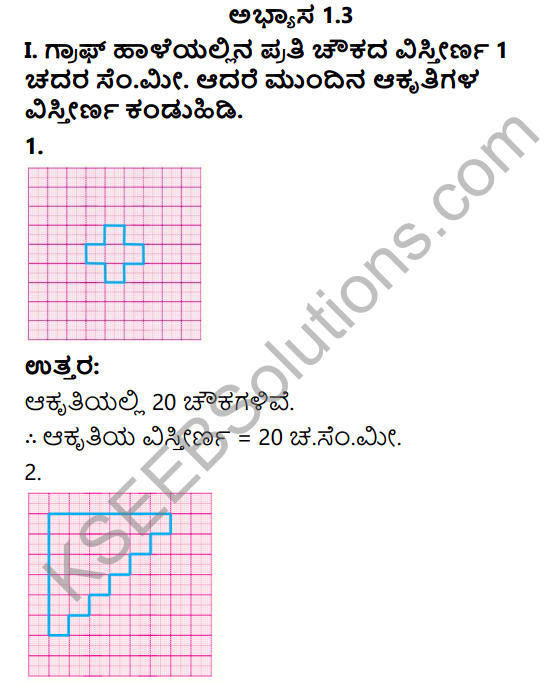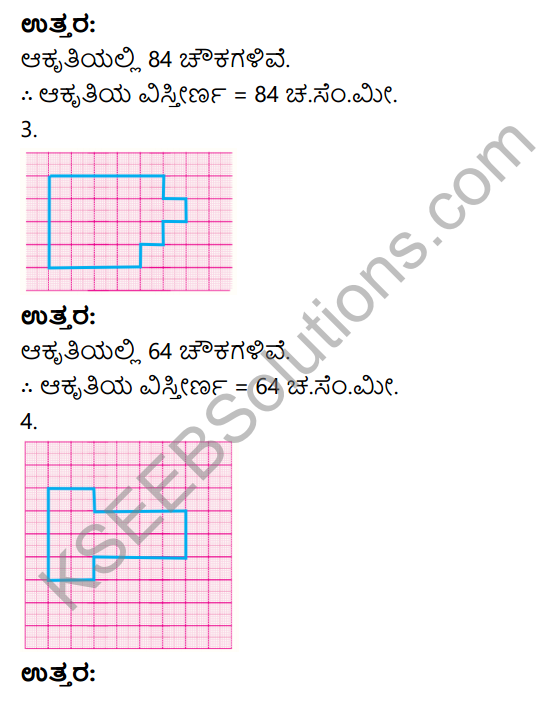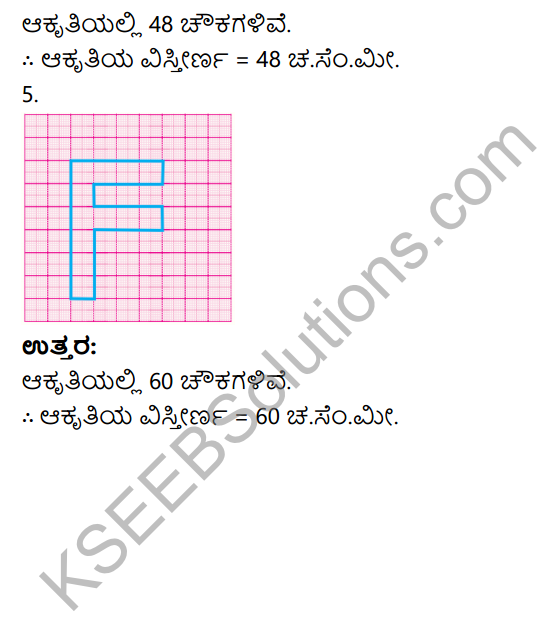# KSEEB Solutions for Class 4 Maths Chapter 1 Perimeter and Area of Simple Geometrical Figures in Kannada

Students can Download Maths Chapter 1 Perimeter and Area of Simple Geometrical Figures Questions and Answers, Notes Pdf, KSEEB Solutions for Class 4 Maths in Kannada helps you to revise the complete Karnataka State Board Syllabus and score more marks in your examinations.

## Karnataka State Syllabus Class 4 Maths Chapter 1 Perimeter and Area of Simple Geometrical Figures in Kannada

### KSEEB Class 4 Maths Perimeter and Area of Simple Geometrical Figures Ex 1.1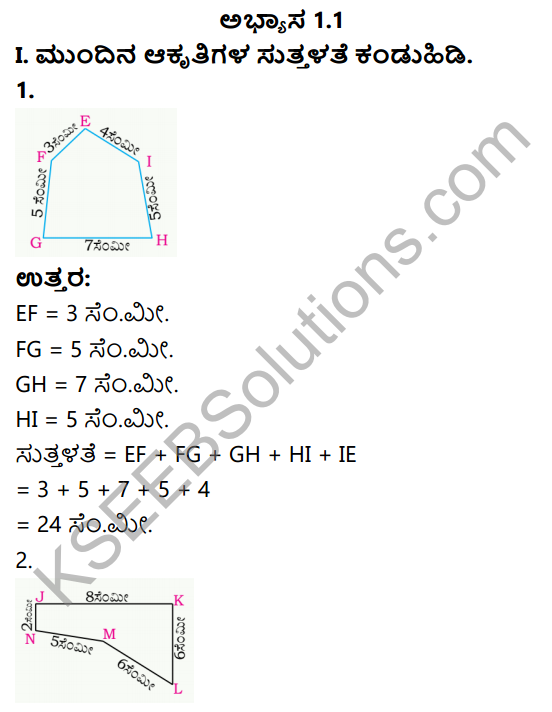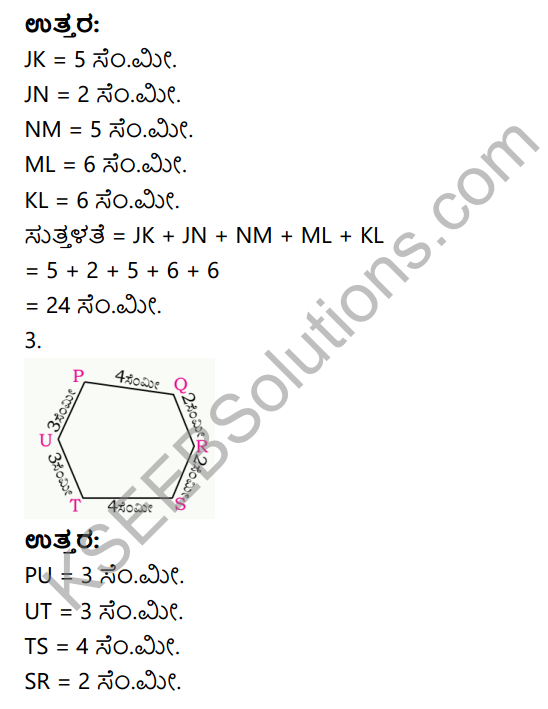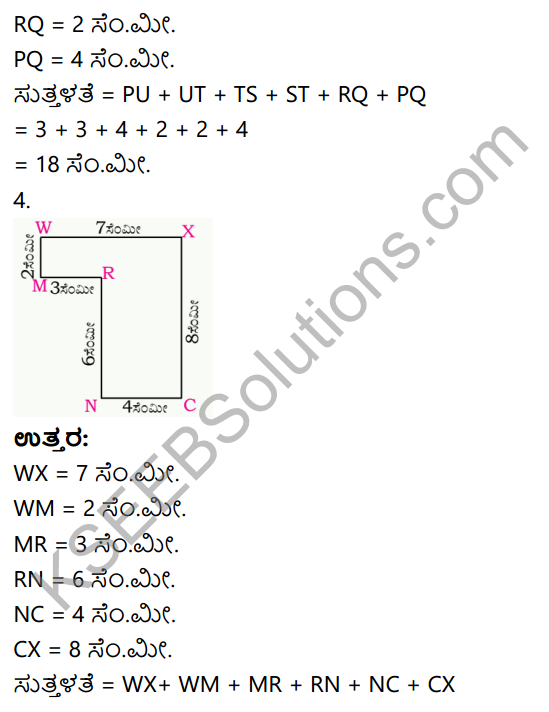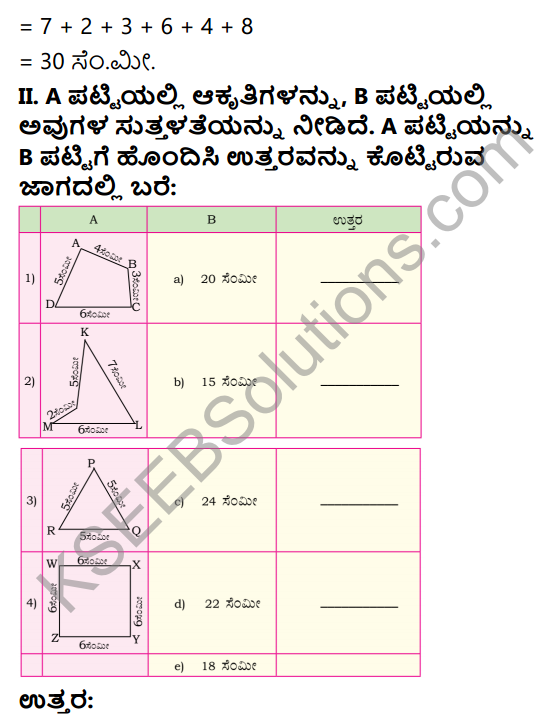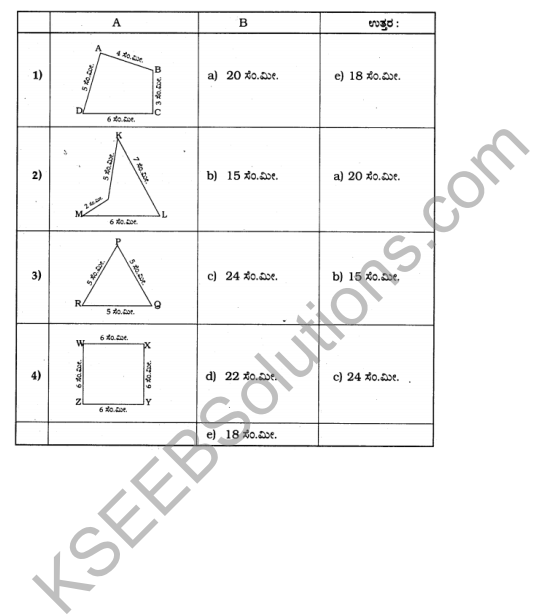### KSEEB Class 4 Maths Perimeter and Area of Simple Geometrical Figures Ex 1.2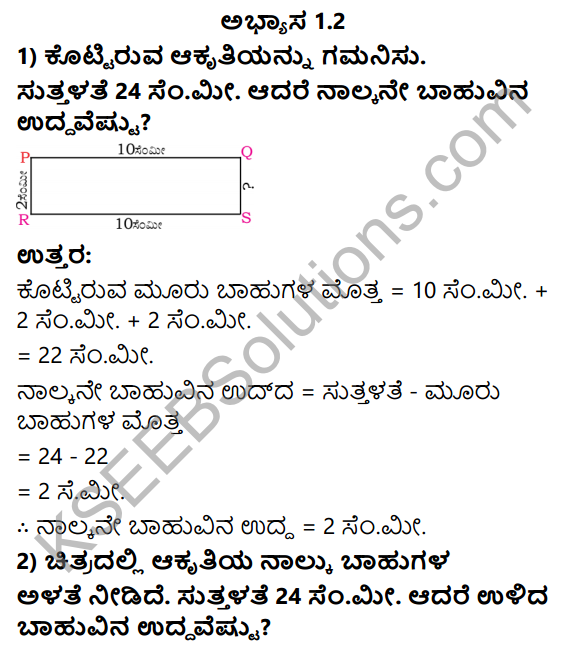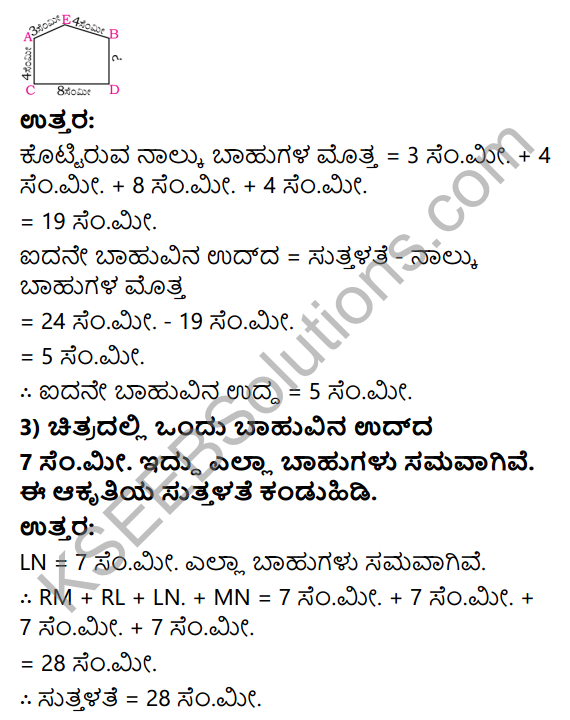### KSEEB Class 4 Maths Perimeter and Area of Simple Geometrical Figures Ex 1.3